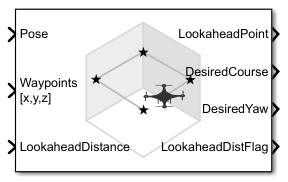Main Content

# Waypoint Follower

Follow waypoints for UAV

• Library:
• UAV Toolbox / Algorithms

•## Description

The Waypoint Follower block follows a set of waypoints for an unmanned aerial vehicle (UAV) using a lookahead point. The block calculates the lookahead point, desired course, and desired yaw given a UAV position, a set of waypoints and a lookahead distance. Specify a set of waypoints and tune the lookahead distance and transition radius parameters for navigating the waypoints. The block supports both multirotor and fixed-wing UAV types.

## Ports

### Input

expand all

Current UAV pose, specified as a `[x y z chi]` vector. This pose is used to calculate the lookahead point based on the input to the LookaheadDistance port. `[x y z]` is the current position in meters. `chi` is the current course in radians.

Example: `[0.5;1.75;-2.5;pi]`

Data Types: `single` | `double`

Set of waypoints for the UAV to follow, specified as a matrix with number of rows, n, equal to the number of waypoints. The number of columns depend on the Show Yaw input variable and the Transition radius source parameter.

Each row in the matrix has the first three elements as an ```[x y z]``` position in the sequence of waypoints.

If Show Yaw input variable is checked, specify the desired yaw angle, `yaw`, as the fourth element in radians.

If Show Yaw input variable is unchecked, and Transition radius source is `external`, the transition radius is the fourth element of the vector in meters.

If Show Yaw input variable is checked, and Transition radius source is `external`, the transition radius is the fifth element of the vector in meters.

The block display updates as the size of the waypoint matrix changes.

Data Types: `single` | `double`

Lookahead distance along the path, specified as a positive numeric scalar in meters.

Data Types: `single` | `double`

### Output

expand all

Lookahead point on path, returned as an `[x y z]` position vector in meters.

Data Types: `single` | `double`

Desired course, returned as numeric scalar in radians in the range of `[-pi, pi]`. The UAV course is the angle of direction of the velocity vector relative to north measured in radians. For fixed-wing type UAV, the values of desired course and desired yaw are equal.

Data Types: `single` | `double`

Desired yaw, returned as numeric scalar in radians in the range of ```[-pi, pi]```. The UAV yaw is the forward direction of the UAV regardless of the velocity vector relative to north measured in radians. The desired yaw is computed using linear interpolation between the yaw angle for each waypoint. For fixed-wing type UAV, the values of desired course and desired yaw are equal.

Data Types: `single` | `double`

Lookahead distance flag, returned as `0` or `1`. `0` indicates lookahead distance is not saturated, `1` indicates lookahead distance is saturated to minimum lookahead distance value specified.

Data Types: `uint8`

Cross track error from UAV position to path, returned as a positive numeric scalar in meters. The error measures the perpendicular distance from the UAV position to the closest point on the path.

#### Dependencies

This port is only visible if Show CrossTrackError output port is checked.

Data Types: `single` | `double`

Status of waypoint navigation, returned as `0` or `1`. When the follower has navigated all waypoints, the block outputs `1`. Otherwise, the block outputs `0`.

#### Dependencies

This port is only visible if Show UAV Status output port is checked.

## Parameters

expand all

Type of UAV, specified as either `fixed-wing` or `multirotor`.

This parameter is non-tunable.

Waypoint start behavior, specified as either `first` or `closest`.

When set to `first`, the UAV flies to the first path segment between waypoints. If the set of waypoints input in `Waypoints` changes, the UAV restarts at the first path segment.

When set to `closest`, the UAV flies to the closest path segment between waypoints. When the waypoints input changes, the UAV recalculates the closest path segment.

This parameter is non-tunable.

Source of transition radius, specified as either `internal` or `external`. If specified as `internal`, the transition radius for each waypoint is set using the Transition radius (r) parameter in the block mask. If specified as `external`, specify each waypoints transition radius independently using the input from the Waypoints port.

When the UAV is within the transition radius, the block transitions to following the next path segment between waypoints.

This parameter is non-tunable.

Transition radius for waypoints, specified as a positive numeric scalar in meters.

When the UAV is within the transition radius, the block transitions to following the next path segment between waypoints.

This parameter is non-tunable.

Minimum lookahead distance, specified as a positive numeric scalar in meters.

When input to the LookaheadDistance port is less than the minimum lookahead distance, the LookaheadDistFlag is returned as `1` and the lookahead distance value is specified as the value of minimum lookahead distance.

This parameter is non-tunable.

Accept yaw inputs for waypoints when selected. If selected, the Waypoints input accepts yaw inputs for each waypoint.

Output cross track error from the CrossTrackError port.

This parameter is non-tunable.

Output UAV waypoint status from the Status port.

This parameter is non-tunable.

• `Interpreted execution` — Simulate model using the MATLAB® interpreter. This option shortens startup time but has a slower simulation speed than `Code generation`. In this mode, you can debug the source code of the block.

• `Code generation` — Simulate model using generated C code. The first time you run a simulation, Simulink® generates C code for the block. The C code is reused for subsequent simulations, as long as the model does not change. This option requires additional startup time but the speed of the subsequent simulations is comparable to `Interpreted execution`.

This parameter is non-tunable.

Tunable: No

## More About

expand all

 Park, Sanghyuk, John Deyst, and Jonathan How. "A New Nonlinear Guidance Logic for Trajectory Tracking." AIAA Guidance, Navigation, and Control Conference and Exhibit, 2004.

## SupportGet trial now